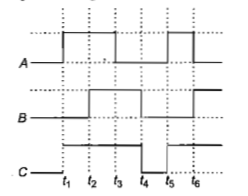The figure shows a logic circuit with two inputs A and B and the output C.The voltage wave forms across A, B and C are as given.The logic circuit gate is(a) OR gate                                          (b) NOR gate

(c) AND gate                                        (d) NAND gate

From the given woveforms, the following truth table can be made

Inputs                   Output

A                   B                       C

0                   0                        0

1                   0                        1

1                   1                        1

0                   1                        1

This truth table is required to 'OR' gate. So, logic circuit gate is OR gate.

Note  The logic of the OR gate is if any of the input is one then output is one.

Difficulty Level:

• 59%
• 15%
• 20%
• 8%